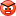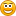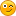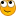Mandarine: original request and ideas676

Hi all

Anyone could make this EA?

Err:= (tPrLong=0) or (tPrShort=0);

a:=0.8;

e1:=Mov(price,Periods,E);

e2:=Mov(e1,Periods,E);

e3:=Mov(e2,Periods,E);

e4:=Mov(e3,Periods,E);

e5:=Mov(e4,Periods,E);

e6:=Mov(e5,Periods,E);

c1:=-a*a*a;

c2:=3*a*a+3*a*a*a;

c3:=-6*a*a-3*a-3*a*a*a;

c4:=1+3*a+a*a*a+3*a*a;

T3MA:=c1*e6+c2*e5+c3*e4+c4*e3;

{Set Moving Averages}

MALong := Mov(Open,tPrLong,mtLong);

MAShort := Mov(Close,tPrShort,mtShort);

{Show Trend Up and Down}

UpTrend:=MAShort>MALong;

DownTrend:=MALong>MAShort;

{Declare variables}

flag1:= If(Prev>0,Prev,0);

shortflag1:= If(Prev>0,Prev,0);

LongPositionFlag:= If(Prev>0,Prev,0);

ShortPositionFlag:= If(Prev>0,Prev,0);

{Signal Long entry if fast MA crosses above slow MA by xamt}

Short:=Cross(MALong,MAShort)And MAShort<T3MA;

{Signal Short entry if fast MA crosses below slow MA by xamt}

Long:=Cross(MAShort,MALong)And MAShort>T3MA;

{Signal close of Long position if fast MA crosses below slow MA+yamt}

Shortstop:=Cross(MAShort,MALong);

{Signal close of short position if fast MA crosses above slow MA-yamt}

LongStop:=Cross(MALong,MAShort);

{Set flag to 1 if long signal detected and set to 0 if close of long position detected}

flag:= if(Long=1,1,

if(Longstop=1,0,prev));

{Set shortflag to 1 if short signal detected and set to 0 if close of short position detected}

Shortflag:= if(short=1,1,

if(ShortStop=1,0,prev));

{Enter long position on long signal only if long position not already open}

longenter:= if((ref(flag,-1)=0 And ref(flag1,-1)=0) And Long=1,1,0);

{Enter short position on short signal only if short position not already open}

Shortenter:= if(ref(Shortflag,-1)=0 And ref(shortflag1,-1)=0 And Short=1,1,0);

{Assume entry price is the closing price if position is entered}

Entryprice:= If(longenter =1,C,Prev);

EntryPriceShort:= If (shortenter=1,C,Prev);

{Set trailing stop TrailAmt below entry price for long position and adjust upwards if price moves up.}

TrailStop:= If (C>EntryPrice, max(C-TrailAmt,Prev),

If (C<EntryPrice, max(EntryPrice-TrailAmt,Prev),

If(C=EntryPrice And LongEnter =1,EntryPrice-TrailAmt,Prev)));

{Set trailStopShort TrailAmt above entry price for short position and adjust downwards if price moves down.}

TrailStopShort:= If (C<EntryPriceShort, min(C+TrailAmt,Prev),

If (C>EntryPriceShort, min(EntryPriceShort+TrailAmt,Prev),

If(C=EntryPriceShort And ShortEnter =1,EntryPriceShort+TrailAmt,Prev)));

{Signal close of Long position if Trailstop hit}

LongstopTrail:= if(c<=TrailStop,1,0);

{Signal close of Short position if TrailstopShort hit}

ShortstopTrail:= if(c>=TrailStopShort,1,0);

{Set flag1 to 1 if long signal detected and set to 0 if trailing stop of long position detected}

flag1:= if(Long=1,1,

if(LongstopTrail=1,0,prev));

{Set flag1 to 1 if short signal detected and set to 0 if trailing stop of short position detected}

shortflag1:= if(short=1,1,

if(ShortstopTrail=1,0,prev));

{Signal close of long position if close detected only if long position open}

SignalLongexit:= If (ref(Flag,-1)=1 And ref(Flag1,-1)=1 And LongStop=1,1,

If (ref(Flag,-1)=1 And ref(Flag1,-1)=1 And LongStopTrail=1,1,0));

{Signal close of short position if close detected only if short position open}

SignalShortexit:= If (ref(ShortFlag,-1)=1 And ref(ShortFlag1,-1)=1 And ShortStop=1,1,

If (ref(ShortFlag,-1)=1 And ref(ShortFlag1,-1)=1 And ShortStopTrail=1,1,0));

{Set LongPositionFlag to 1 if long position has been opened and set to 0 if exit of long position signaled.}

LongPositionflag:= if(longenter=1,1,

if(SignalLongexit=1,0,Prev));

{Set ShortPositionFlag to 1 if short position has been opened and set to 0 if exit of short position signaled.}

ShortPositionflag:= if(shortenter=1,1,

if(Signalshortexit=1,0,Prev));1038

hi,

int Err=0;

int tPrLong;

int tPrShort;

if (tPrLong==0 || tPrShort==0) Err=1;

-STOPis this Metastock code ?

Timeframe ?

Pairs ?

or is this Virus or Wurm676

Alex.Piech.FinGeR:
hi,

is this Metastock code ?

Timeframe ?

Pairs ?

or is this Virus or Wurmhi Alex...

Its my system in VT Trader... Its very profitable...:eek:Timeframe: All, but I prefer 1H

Pairs: All

Thanks

Files:
eurusd.jpg 56 kb585

hellkas:
Hi all

Anyone could make this EA?

As far as I can see it is 2EMA crossover system with T3MA confirmation and trailing stop.

Rules are as follows:

- long signal - fast EMA crosses slow EMA up and fast EMA is above T3MA;

- short signal - fast EMA crosses slow EMA down and fast EMA is below T3MA;

- long exit signal - fast EMA crosses slow EMA down;

- short exit signal - fast EMA crosses slow EMA up;

- trailing stop exits.

hellkas, can you confirm?585

hellkas:
hi Alex...

Its my system in VT Trader... Its very profitable...:eek:Timeframe: All, but I prefer 1H

Pairs: All

Thanks

do you have any stats for yoru system?

what values of parameters tPrLong, tPrShort, Periods and what types of fast and slow MA do you use?676

hi Beluck

This system allows pip entry/exit filters to be applied to a MA Cross System that signals below a defined value are ignored. The system includes a trailing stoploss. The signal is a cross of a long MA of the open and a short MA of the close.

Trailing Stop Pips

Type: Float

0,0020

tPrLong:

Type: Integer

20

Exponential

tPrShort:

Type: Integer

5

Exponential

Price: Close

Periods:

Type: Integer

51038

@Beluck make you this EA ?

or i make ?676

Hi Alex...

Isnt mine, but I use it.. Its Great...

If its yours, Im sorry and thanks...

Could you help me?

hellkkas1038

Hi hellkkas...

ok i make EA for MT 4676

Alex.Piech.FinGeR:
Hi hellkkas...

ok i make EA for MT 4Ok my friend... Thanks very much...But where it is?1038

i begin NOW convertok?